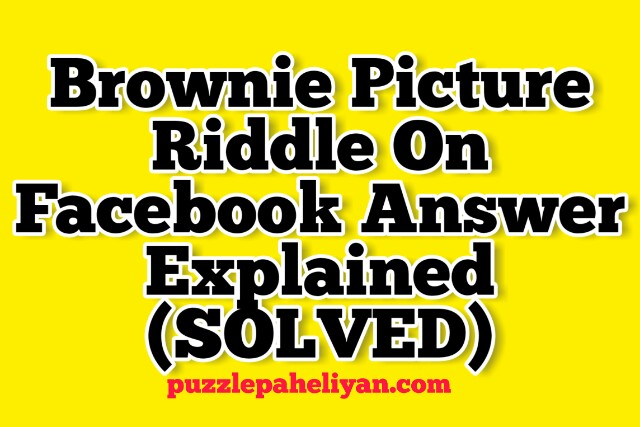# Category Archives: Picture Puzzles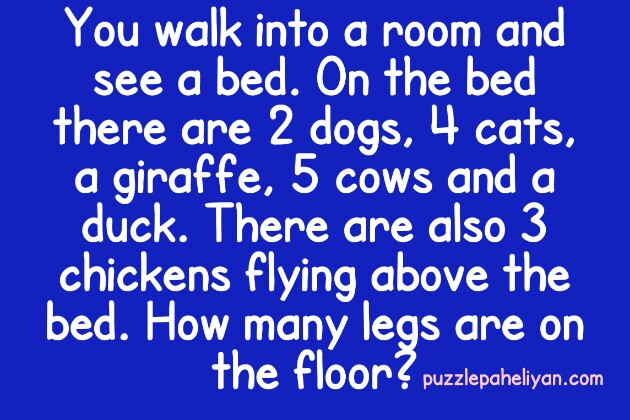You walk into your room to your bed; Riddle is an exciting and logical Riddle. The best part of this Riddle is it has different answers, and every time you ask this Riddle to someone, they will come up with different solutions.

Riddle: “You walk into a room and see a bed there and On the bed, there are (two)2-dogs, four cats, a giraffe, 5 cows, and a duck there. There are also 3 chickens flying above the bed which you see. How many legs are on the floor?”

Answer: You walk into a room, and on the bed, Riddle’s response is 6 or 2 legs.

The first thing which is to be considered is that Riddle asks for the legs which are on the floor and none of the animals are on the floor so we will not count the legs of the animal.
You entered the room so your legs are on the floor and the four legs of the bed. So in total, there are six legs, or if we ignore the legs of the bed, then the answer is six legs.

# A boy was playing with a book riddle answer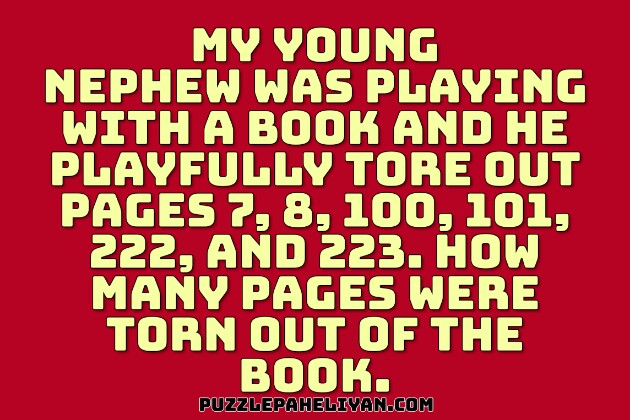A boy was playing with a book riddle is one of the best logic riddles of all time, and it’s been trending on the internet, and people are talking about it. The Riddle test your logic skills and your proper concentration power.

Riddle: “My nephew has a book and he was playing with a book, and he playfully tore out some of the pages which are as follows 7, 8, 100, 101, 222, and 223. How many pages were torn out from the book? tell”

Answer: A boy was playing with a book and tore out the page’s response is five pages.

Initially, when you see this question, You would think that there are only 6 Page numbers are written here, so the answer should be six, but it’s not.

Let me explain to you how every single page of a book has two numbers written on each side like odd and even because the number will start from an odd number like 1.

1st page will have 7 and 8 numbers on it, 2nd page will have 99 and 100, 3rd page will have 101 and 102, 4th page will have 221 and 222, and the 5th page will have 223 and 224, which makes it a total of 5 pages.

# I am a box that holds keys riddle answer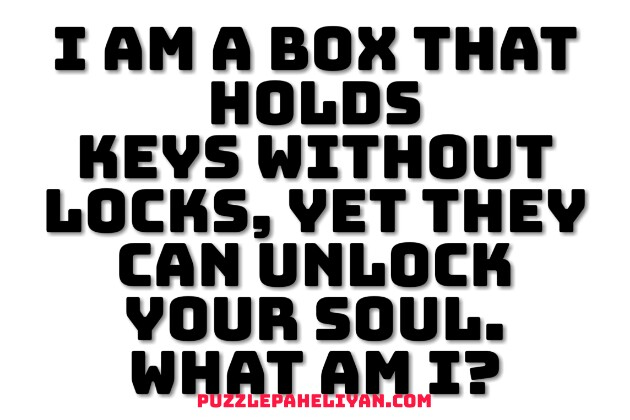I am a box that holds keys riddle says about a thing which is a kind of box and has keys inside them but doesn’t have any locks. But still, it can unlock your soul with its keys.

Riddle: “I am a box that holds many keys of 2 colors without any locks yet they can unlock your soul What am I?”

# A monkey a squirrel and a bird riddle answer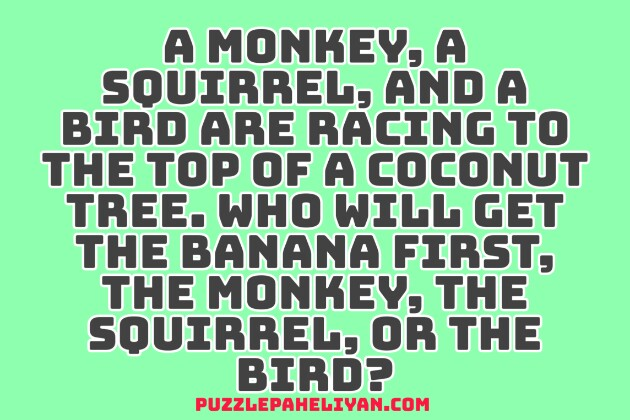A monkey, a squirrel, and a bird are racing to the top of a coconut tree Riddle is one of the best riddles of all time. The Riddle is not about the answer; it’s about the concentration and your focus on understanding things quickly.

This Riddle is a little tricky, and the answer lies in the question itself, so reading carefully.

Riddle: “A monkey, a squirrel, and a bird came together for racing to the top of a coconut tree and to see Who will get the banana first, the monkey, the squirrel, or the bird? tell”

Answer: The correct answer is no one will get the banana. Because they are racing on a coconut tree to get a banana.

# Puzzles with Answers – Maths puzzles Questions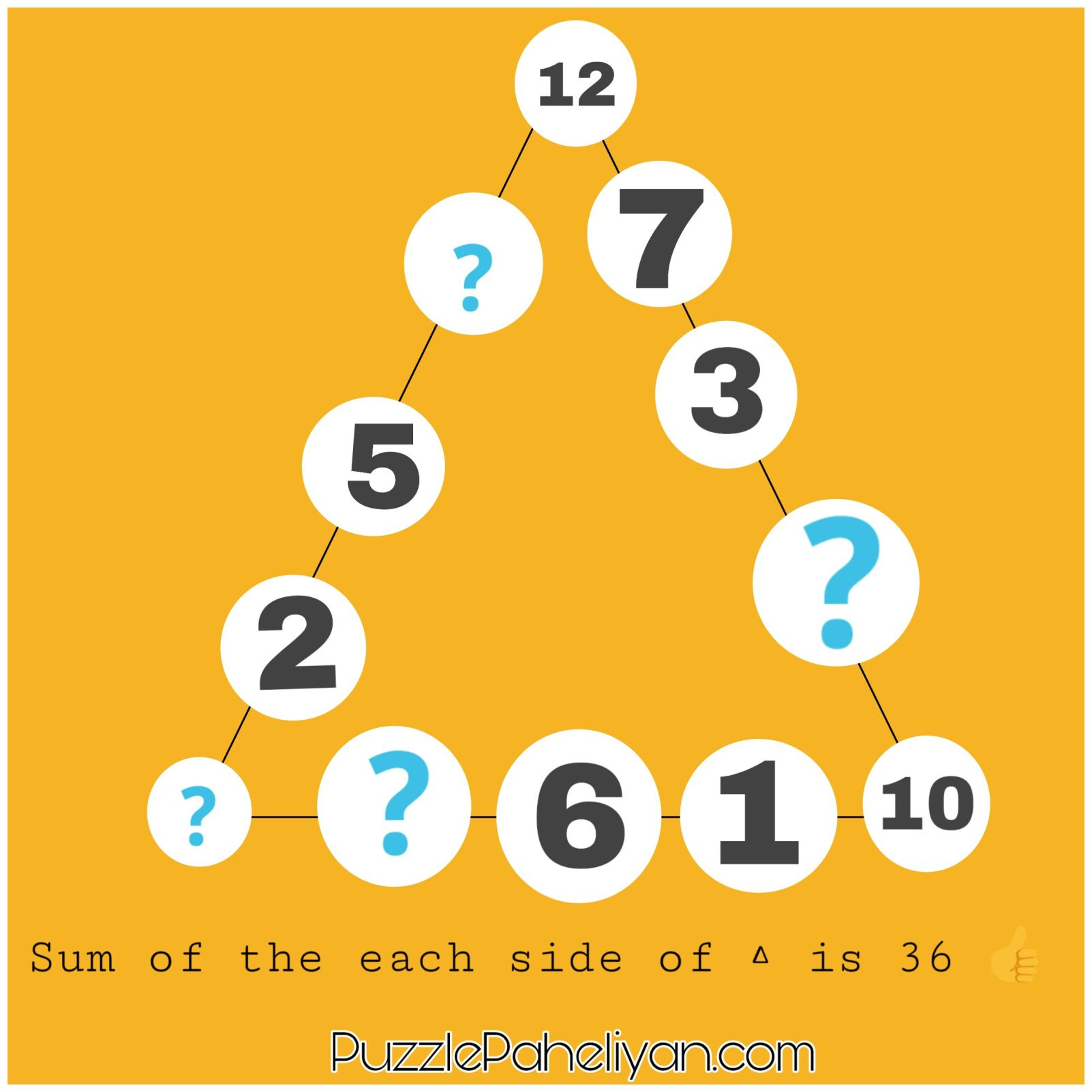Puzzles are a fascinating subject that everyone likes, and everyone wants to solve them. People take them as a challenge and find answers to their Puzzles questions. Puzzles can help to exercise our brain, so I have brought you some entertainment, and a little difficulty Maths puzzles. Items that you can try to solve. Answers to all the riddles are given in the last.

## List of Puzzles Related To Maths

1. In this first question, you have to tell the next number which will comes in the series of 1, 4, 9, 16, 25, 36. find the following figure?

2. In this Puzzle, you have to put the numbers from 1 to 12 in these circles so that the sum of the sides of each triangle is equal to 36. Some figures have already been given in the question below and keep in mind that the same number cannot be repeated. It can only be used once.

3. Deepak works in a company where he earns Rs.350 per hour. He works only for five days a week and works only for 5 hours every day, and he will work for the next five weeks continuously.
1) How much money will Deepak make in a week?
2) How much money did Deepak earn in total?
4. In this Maths Puzzle below, shapes are added and give some value. Find the value of the triangle by looking at the picture below.

5. Mr.Puzzle Man buys a smart TV for \$ 255. When he goes to the check out counter, Mr.Puzzle  gets a further discount of 10%. So you have to tell me how much will the Smart TV cost after getting the refund?
6. In this Addition Problem, letter B and C denote different numbers. You have to find the value of B and C by looking at the picture below.

7. Look at the picture carefully and answer the pie puzzle. There are many numbers written in the circle below from which 1 number is missing. You have to find the same missing number in the pie puzzle.

## Answers for Puzzles in Hindi:

1. The next number in the series is 49.
2. The correct answer is 12,7,3,4,10,1,6,11,8,2,5,9 which makes the sides equal to 36.
3. The correct answer is Deepak earns Rs 8750. calculation: 350 * 5 hour = 1750 1750 * 5 = Rs 8750
4. The value of the triangle is 10.
5. Mr.Puzzle will get 225 \$ discount.  calculation: 10% discount will be 1/10 of price, is 25 \$ 250-25 = 225 \$
6. The value of B and C will be 8 and 7, respectively.
7. Answer is 10, opposite add 21, 15 + 6 = 21

If you liked these puzzles, you can check out more on our website and do share it with your friends. You can also read our more articles on puzzles, riddles, and more.

# Tricky Maths Puzzles with Answers [BEST]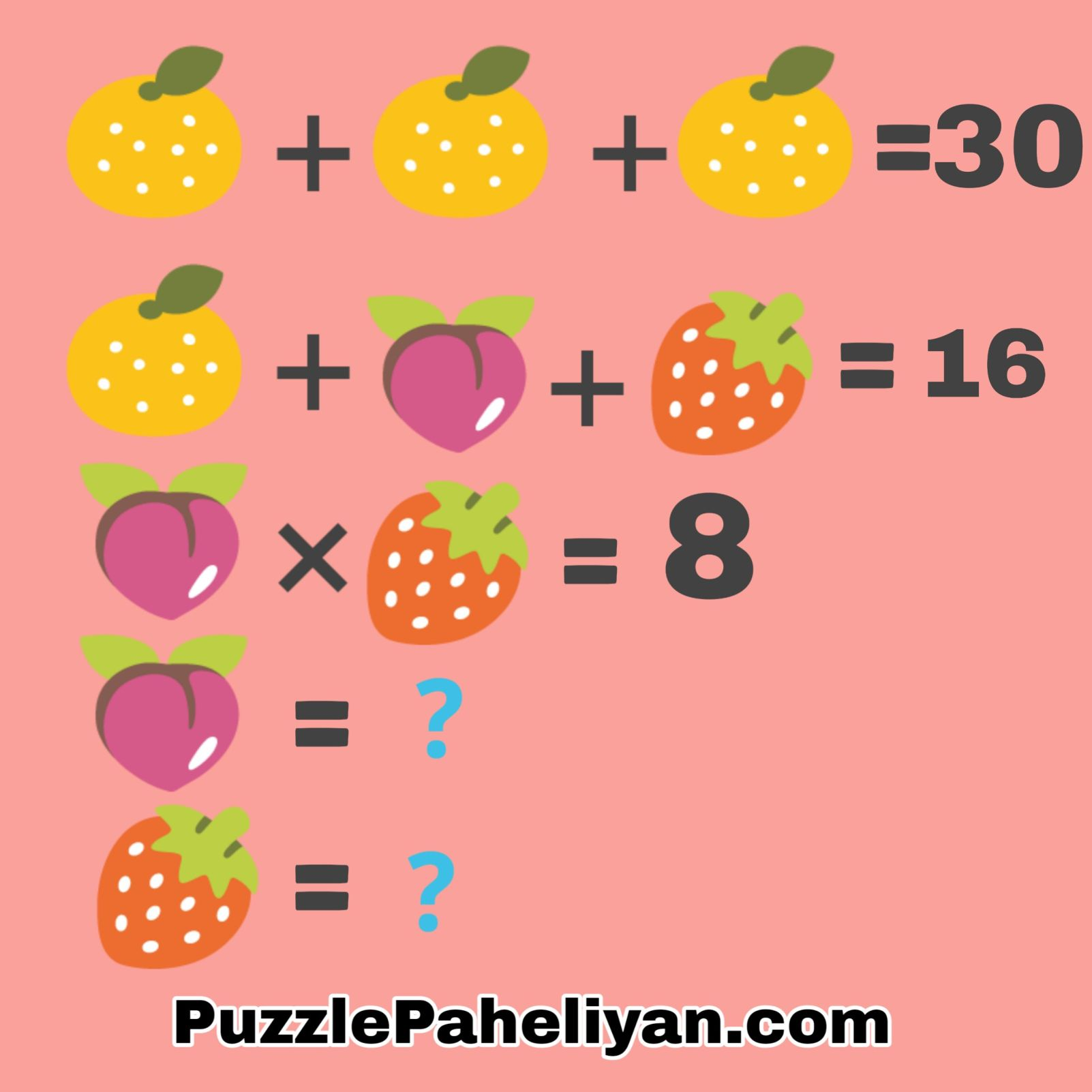I have brought you some best and simple Tricky Maths Puzzles with Answers for you. It also has some puzzles related to maths and WhatsApp puzzle questions and answers, which you can also share with your friends.

All these Puzzles are connected to Maths and simple to answer, and the images of puzzles are also given below so that you can understand then easily. This is a relatively simple addition and subtraction puzzle that even young children can solve.

## List of Maths Puzzle Questions

### Puzzle 1

In Puzzle 1, you are given three different emoji. In which, adding the first three emojis faces gives number 15 and adding a face, heart, letter emoji gives numbers 30. You have to find how many numbers will come by multiply the letter and heart emoji.

### Puzzle 2

This puzzle is also the same as the previous question, but it has a small number difference, and this is a bit tricky than the last question, but if you look at it carefully, you will be able to answer it very quickly.
In this emoji puzzle, you have been given different numbers of fruits, so look carefully and understanding it and find the value of Peach and Strawberry.

### Puzzle 3

In the third puzzle, you get 60 by adding three Lion emoji and 24 by adding one Lion, Tiger, and a Fox. If Tiger and Fox get multiply, you get three, so calculate the value of Tiger and Fox emoji.

### Puzzle 4

This puzzle is also like the previous puzzle, and the only difference is it contains some special characters in place of emojis.
By adding the first three characters, the number coming off 27, and by adding the other three characters, the name coming off 24, then you have to find the value of the remaining characters.

### Puzzle 5

As you can see in the photo, adding two Unicorn emojis, it gives 25, and if I multiply one Unicorn and Horse emojis, it will be equal to how much.

## Quotes on Puzzle Life

The life of every person who comes into the world is like a puzzle, which has to be solved by that person every moment. There are many such moments in our life where we have to face difficulties and challenges. By moving in the right direction, the person can overcome his problems.

I hope you like the puzzles related to Maths Puzzles and share it with your friends on Facebook, Instagram.

# 7 Maths Logic Puzzles with Answers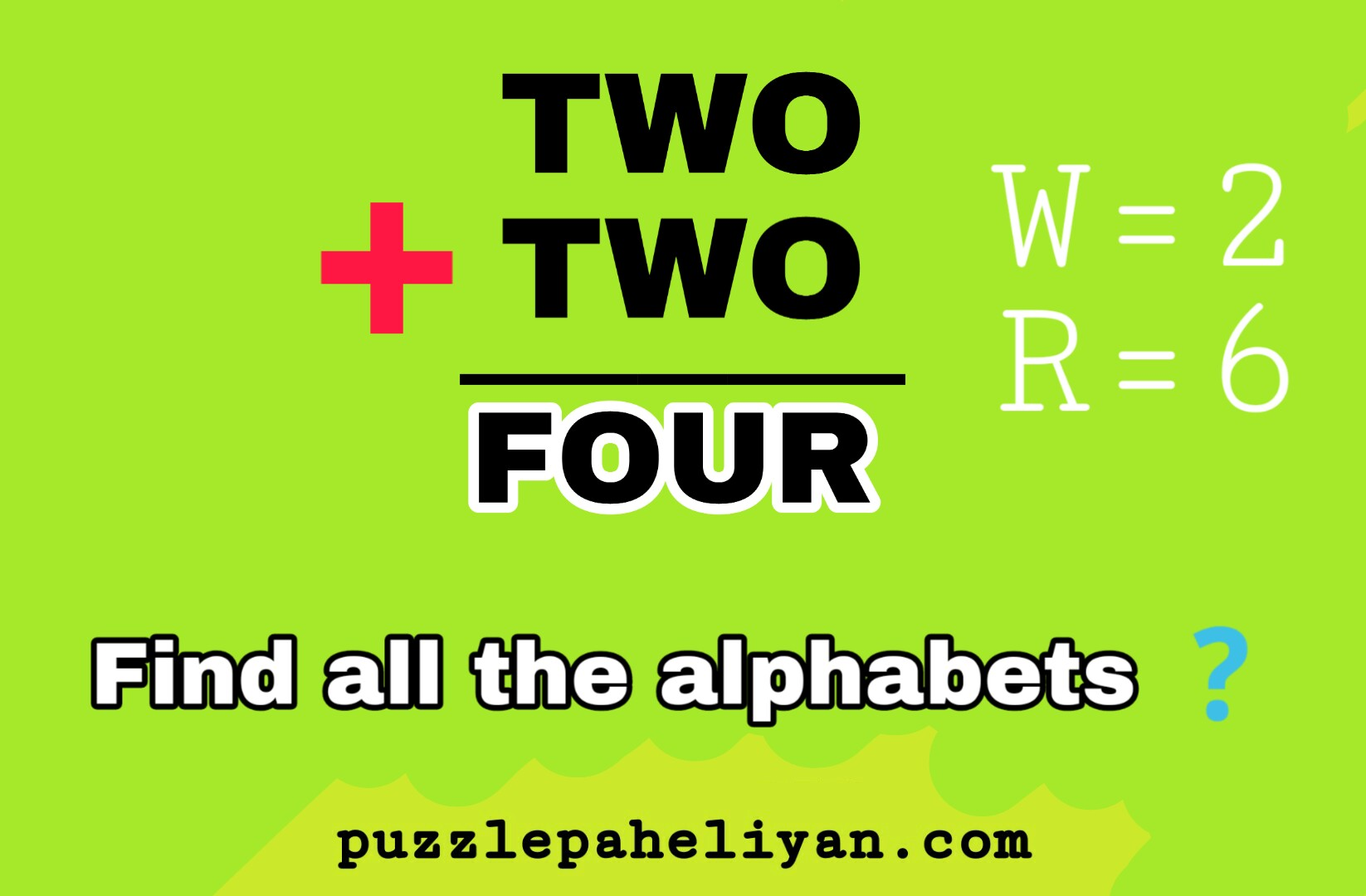Best collection of Maths Logic puzzles with answers, Challenging Puzzles of Marks, and photo maths puzzles for kids. If you are looking for some challenging problems, then you have come to the right article. In this article, there are many different types of Puzzles and Riddles.

Maths logic Puzzles

We have some new maths logic puzzles and some riddles. I have done a lot of research for you and found out some challenging questions related to this topic. Along with all the questions, I have also given different images, seeing which you can find the answer to the problem, and if you like the issue, then you can share it among your friends and ask them this question too.

## Maths Puzzles Questions

1. In the photo below, a logic pattern is being created, which you have to find by looking at different numbers, and you have to tell what should be the last number of these boxes according to the puzzle grid?

2. Can you find the missing number puzzle? Look at the tables given below and apply logic questing and explain what number should come in the third box.

3. Every number in each square has some kind of relationship with each other, which is similar to the other six boxes. Find the Missing Number and find the relationship between these numbers as well?

4. This is an additional question problem in which A and B are any two-digit letters. Keep in mind B letter is not zero, can you tell me the number for A and B?

5. Below is a very tricky puzzle. Some letters indicate different numbers. You can choose up to zero to 9 Digits and remember that every letter can be a digit number and can also be zero.
hint: W = 2 and R = 6

6. Looking at the first three circles, can you tell the patter and number relationship how the other four rings are similar?
By looking at the first three circles, you have to find the pattern of the number and find the answer to the fourth circle.

7. Rahul’s sister makes 17 points in her school basketball game, and she has 2 points short of 2 point number and even 3 point shots of number 1 and point shots. And she does not also take any free throws, so tell you how many baskets Rahul’s sister must have done for every point type?

## Answers for the maths puzzles

1. The missing number is 3. answer explanation is this-
• 6×3=18
• 4×9=36
• 7×5=35
• 9×3=21
• 3×8=24
2. The right answer is 24. You have to double the number first number of every rectangle and write in the next column.
3. The missing number is 4. You need to add every 3rd number, and you will get the total sum equal to 12.
4. letter A is six and Letter B is 5
5. Answer is  928+928= 1856. where-
F=1
O=8
R=6
T=9
U=5
W=2
6. The missing number and the correct answer is 3.
7. Four two-point shots and three 3 point shots.

If you love these puzzles, you can also check more articles related to problems on our website. You can also share the articles on social media accounts with your friends to know whether they can give the right answers to these questions.

# Simple Math Puzzle question and answers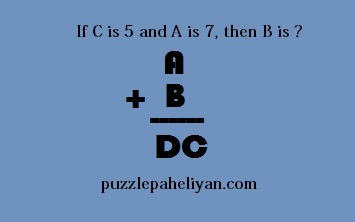In this article, I have brought you an excellent and Simple Math Puzzle questions and answers. The answer to the puzzles is given at the end of the article. You will find a lot of problems, but today I brought a different type of questions for you.

If you enjoy creating your puzzle, then I have brought a perfect example for you so that you can make your puzzle question.

steps: First, write the count from 1 to 9 in a straight line. like this-1, 2, 3, 4 5, 6, 7, 8, 9.
Now you have to set a goal in your mind, and according to your purpose, which you have set in your account (number), place the different symbols in between them and make a total of that.

Now try to use different methods to get 100 in your total answer.

## Math Puzzle questions

1. Out of the four options given below, you have to solve them and tell which option will provide you with seven answers.
• (6 × 5) ÷ 2
• (3 + 4) ÷ 5
• (10−4) + 1 ÷ 1
• (12−5) +2
2. In the picture given below, you can see that in the pyramid, some number is missing. When we add the bottom two Brick, it is equal to the top Brick. Some names have already been given in the picture, and you have to look at all of them and try to find the rest numbers.

3. In this puzzle, you have to find the mystery number.
My hundred or number is equal to half of the number of my teeth. My one-digit number is the same to double my teeth. And none of these three places is number one. What number am I _ _ _?
4. In the math problem given below, A, B, C, D are all single numbers.
And none of these numbers are zero.

If C is five and A is 7, then tell B how much.

Answer: The answers to the riddles are given here. I hope you like the questions, and if you have, then you should share them with your friends on Facebook and WhatsApp.